# The symbol brain processor - optical nerve military news on the Science Wars

From the Z-papers by Herb Zinser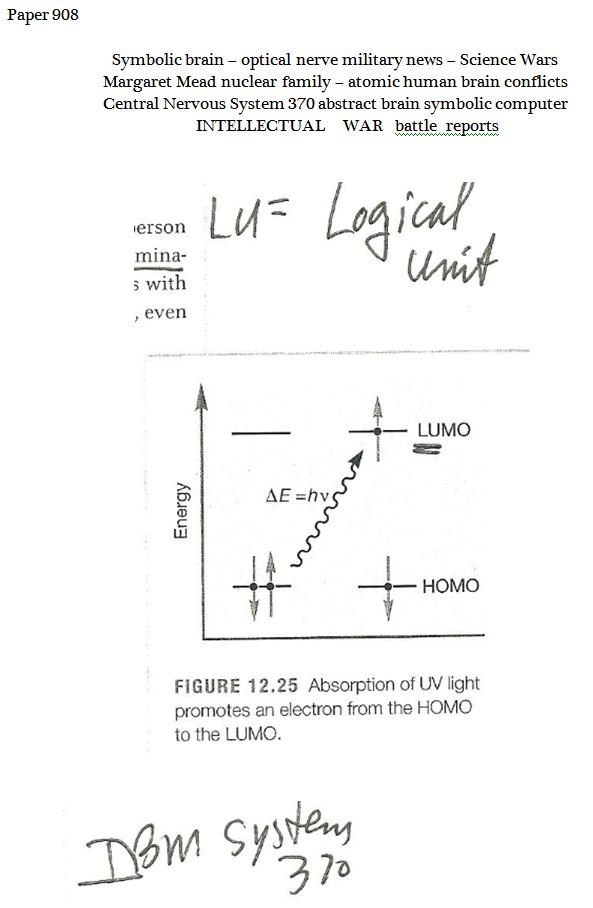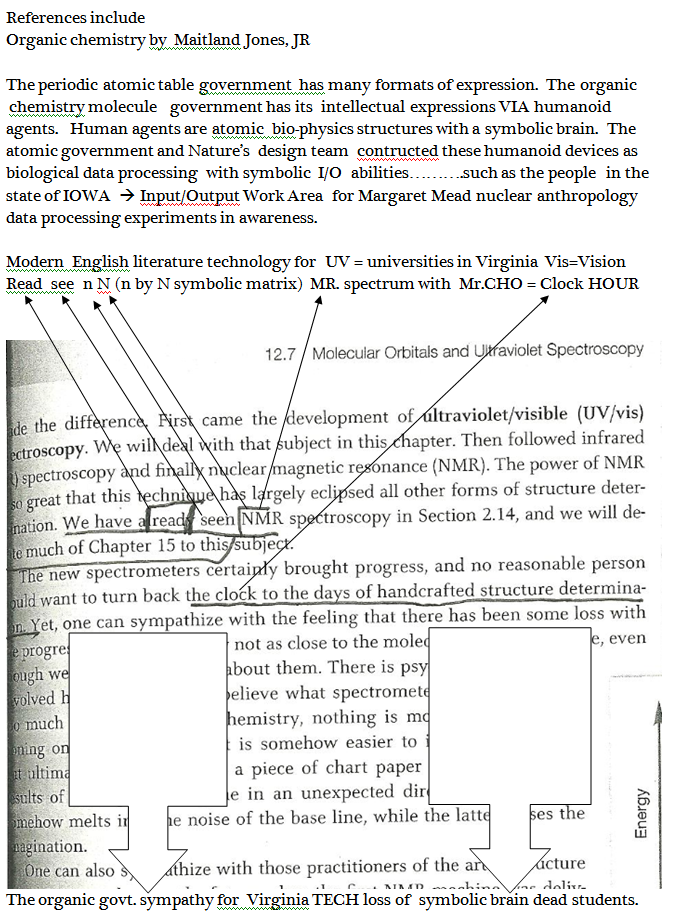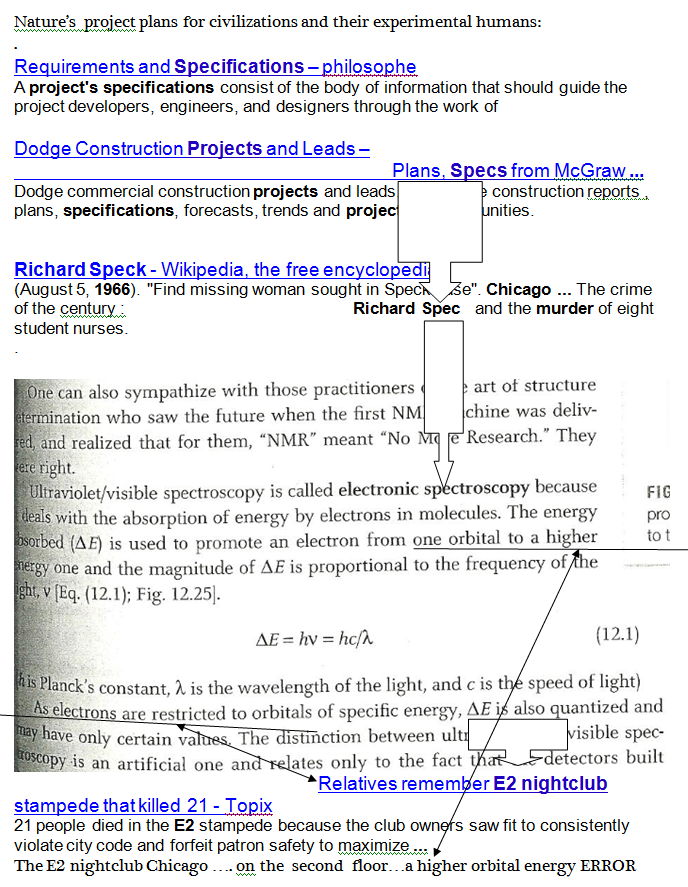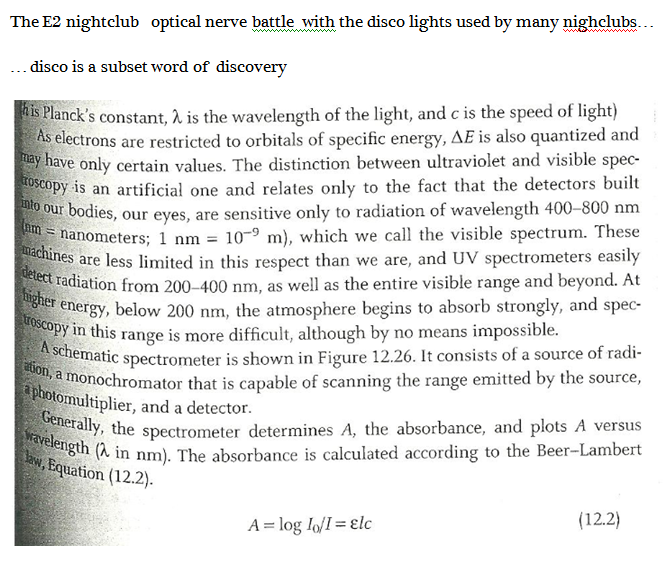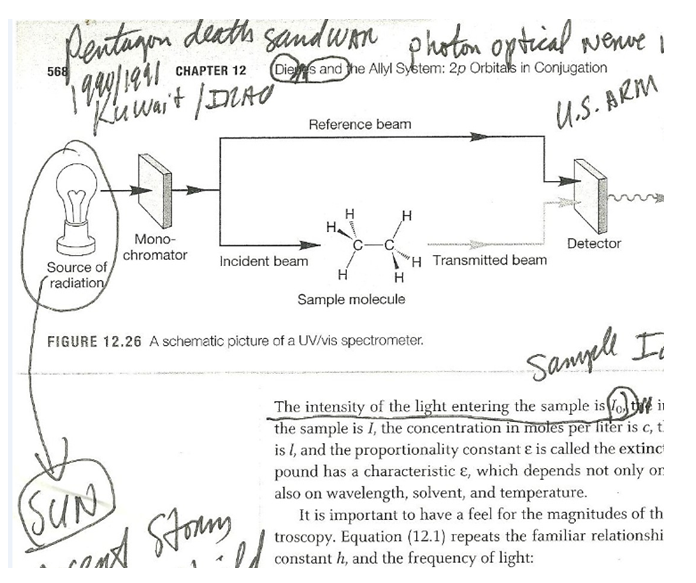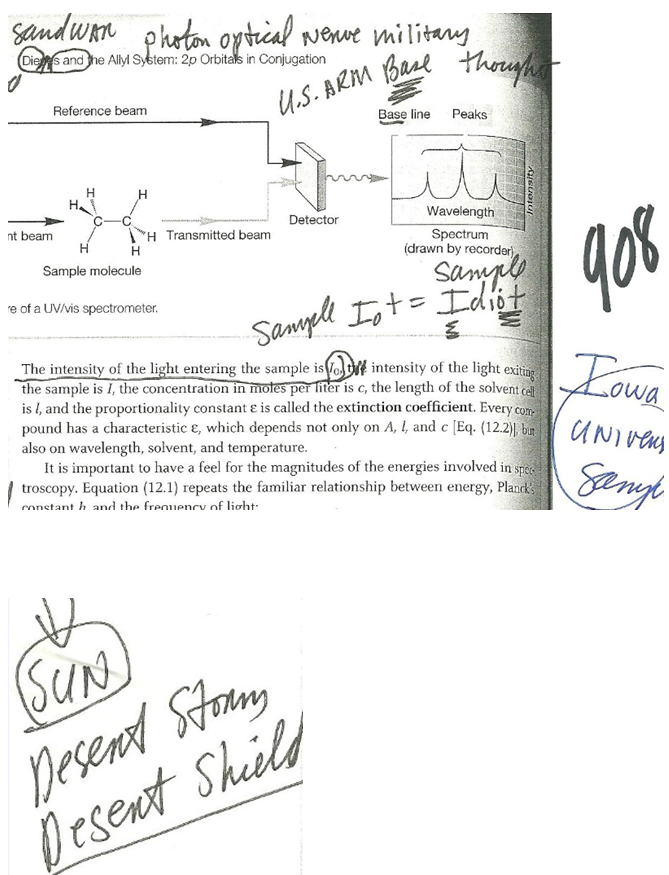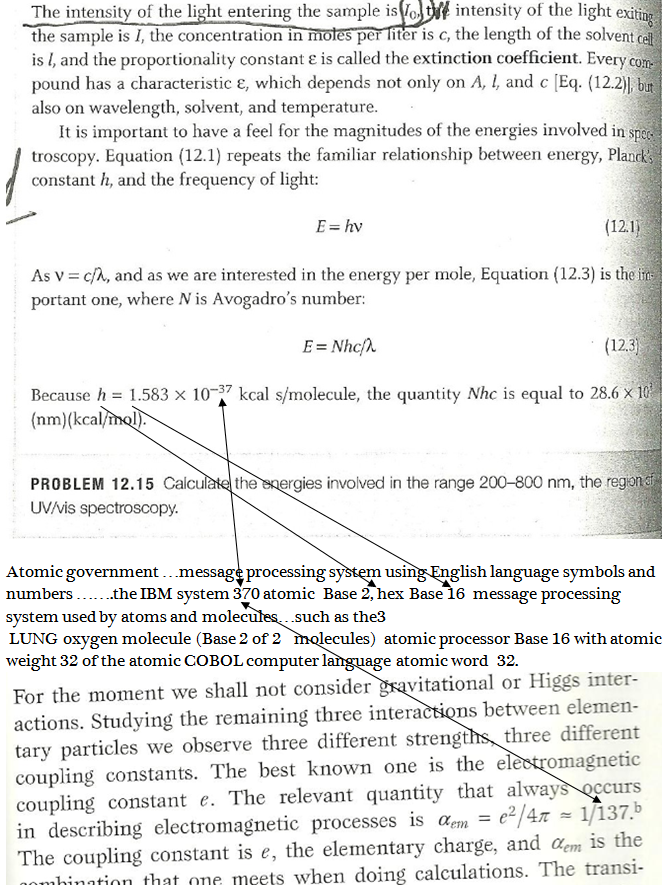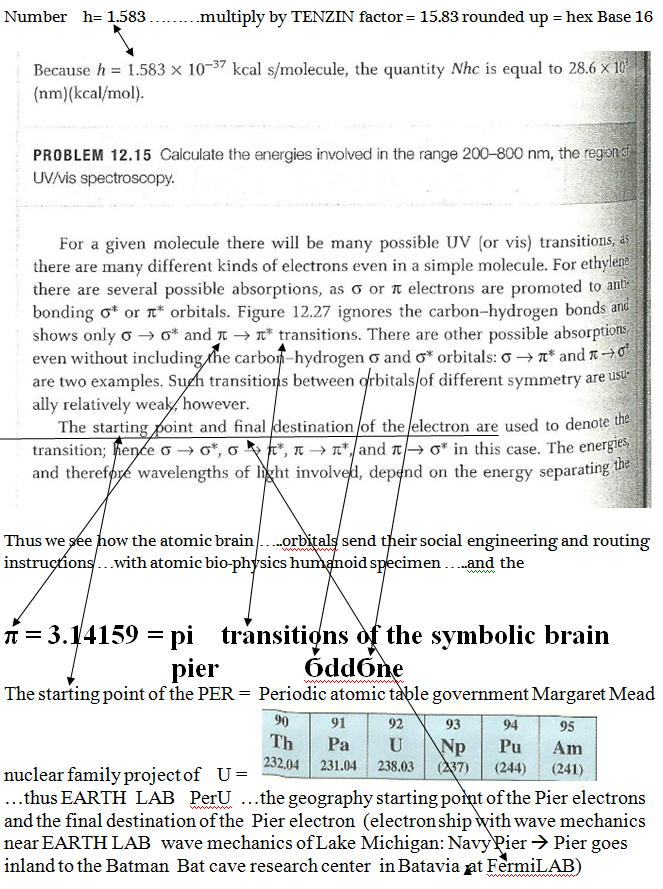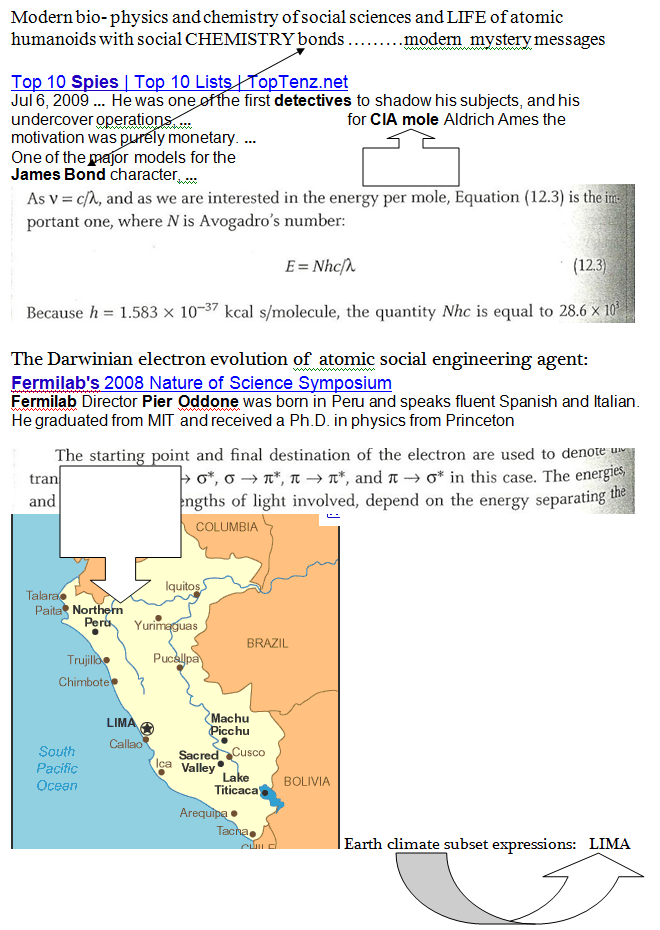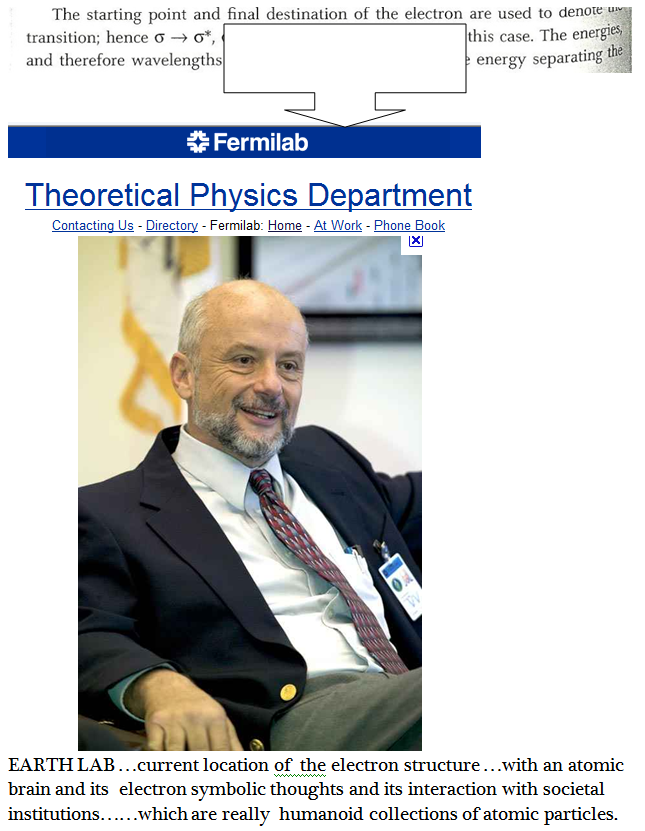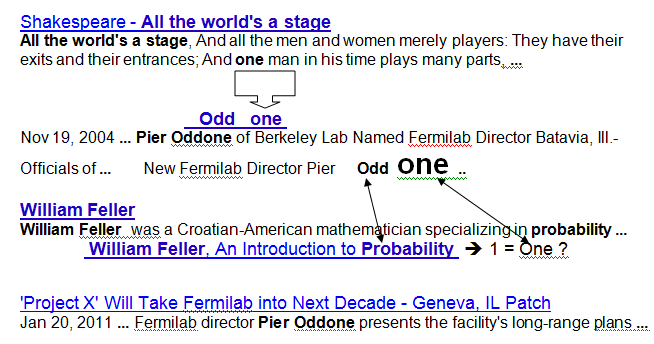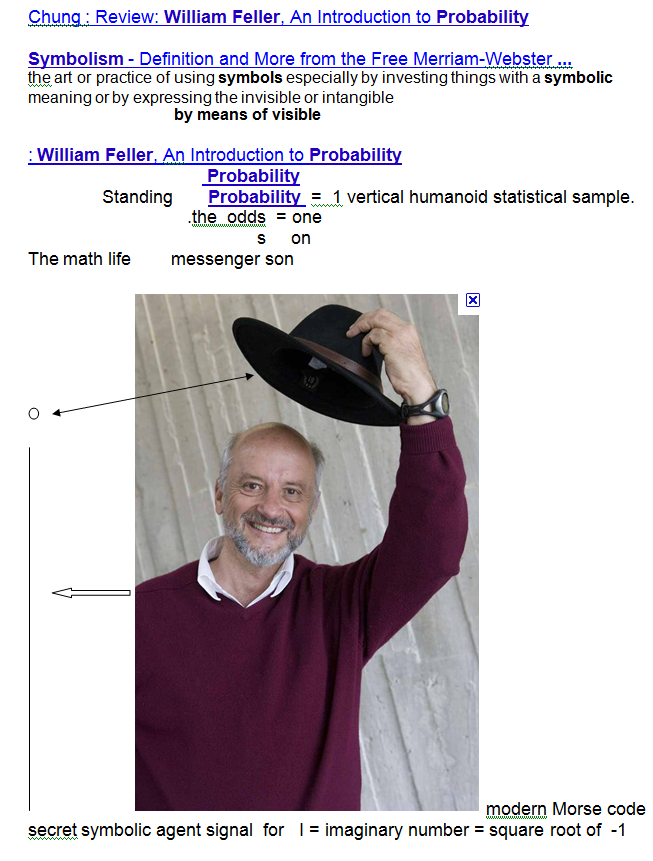Notice his body  language    bio-math signal  .....  for

The imaginary unit or unit imaginary number, denoted as i, is a mathematical concept which extends the real number system to the complex number system , which in turn provides at least one root for every polynomial P(x)     (see algebraic closure and fundamental theorem of algebra). The imaginary unit's core property is that i2 = −1.

There are in fact two complex square roots of −1, namely i and i, just as there are two complex square roots of every other real number, except zero, which has one double square root.

# Imaginary unit

Notice his bio-math name ........   Pier O --> except zero, which has one double square root ...... thus suggesting he is a secret  DOUBLE AGENT for mathematics   ....
working at FermiLAB   near DuPage County   ...... identifier DuPage --> Duplicate  or Double  Page  .................... thus his double square root identity fits right into that mathematical-physics geography region of Landau geo-physics and bio-physics.